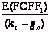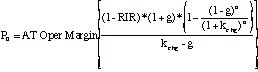Typos that I have found (and I am sure you will find more...)

After 6 books and several thousand pages of manuscript, you would think that I have found the secret to error-free publishing but you would be wrong... Here are the typos I have found so far. What can I say other than "I am sorry"?

 Page number Text as is Corrected text 40 FCFE11 = 15400 (1.06) - \$15,400(1.06)(.40) –(-653) = \$9,142 million Terminal Price =  9,142/(.104-.06) = \$207,764 mil Value of Equity today = 25,461+207764/1.10410 = \$102,708 million Value per share = \$102,708/997.231 = \$102.99 FCFE11 = 15400 (1.06) - \$15,400(1.06)(.40) –(-653) = \$10,447 million Terminal Price = 10447/(.104-.06) = \$237,441 mil Value of Equity today = 25,461+237441/1.10410 = \$113,742million Value per share = \$113,742/997.231 = \$114.06 41 Value of firm with FCFF growing at Constant rate =Value of firm with FCFF growing at Constant rate =71 Cisco is on Y axis; S&P 500 is on X axis Cisco is on X axis; S&P 500 is on Y axis 97 Estimated MV of Motorola Debt = [ Estimated MV of Motorola Debt = \$305[ (Insert annual interest expense before first bracket) 125 The net loss of \$852 million The net loss of \$752 million 147 The arithmetic average growth rate is lower The arithmetic average growth rate is higher 192 If the return on capital is equal to the stable growth rate If the return on capital is equal to the cost of capital 316 EV/EBITDA = (Market Value of Equity – Market Value of Debt – Cash)/EBITDA EV/EBITDA = (Market Value of Equity + Market Value of Debt – Cash)/EBITDA 332I hope you have not bought anything or sold anything because of these typos.